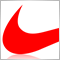# Forex Margin, have I understood it?53

Hey guys quick question here...

How to properly calculate margin?

As far as I understand if you have a 1:100 leverage that is 1% margin required of your lot size. Therfore if you have a lot size of 0.1 (10,000) that is £100. However, when I open certain currencies the margin is sometimes above/less than the calculated £100 - so could you let me know how it is properly calculated? Is it 1% of the lot size * currency. So for example EURUSD @ 1.50. If lot at 10,000 then 10,000*1.50= 15,000 and 1% of 15,000 then margin required for that trade is £150?

I look forward to your replies. thanks.53

wow, does no one know about margin then?4636

If you do a query on Google, you will get lots of info: here is an example from one of the results:

How to Calculate Leverage, Margin, and Pip Values in Forex, with Examples